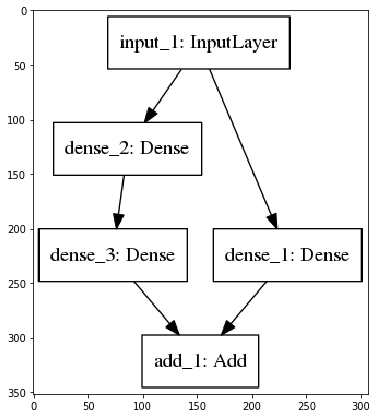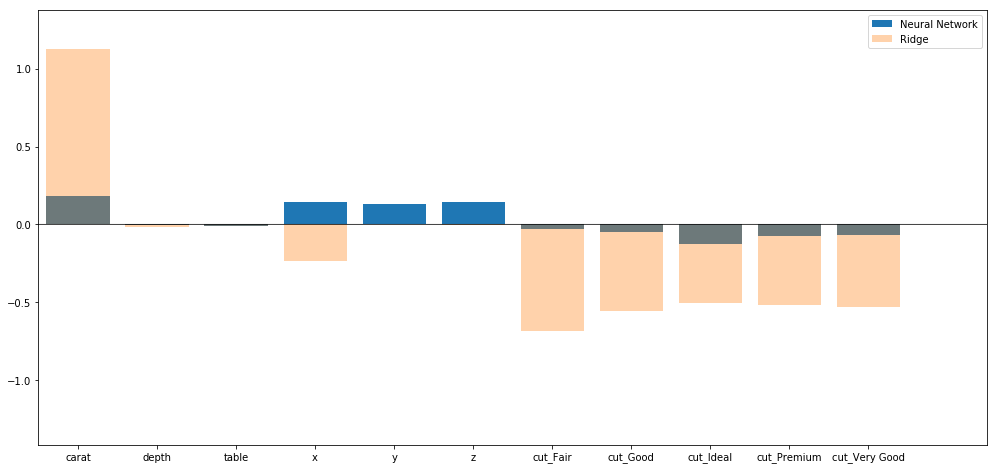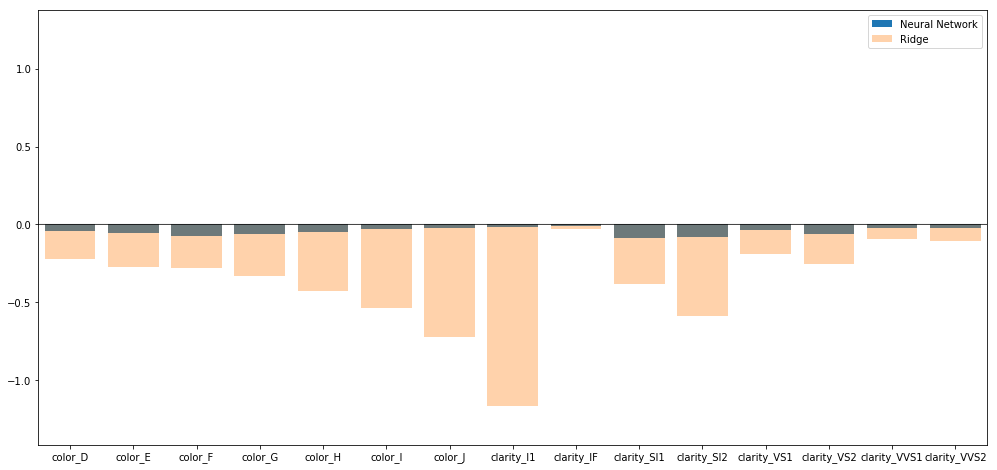## Introduction¶

While the current hype about Deep Learning and Neural Networks is understandable given the vast number of cases where ANNs clearly outperformed every other algorithm, a lot of people think (myself included) that Neural Networks won't be the ONE Machine Learning algorithm that will solve every problem one day. Nevertheless, the Neural Network framework offers a lot of powerful tools that have proven themselves worthy of their popularity. Today I want to present a Feedforward Network based approach that I was having on my mind for some time now and finally managed to test on Kaggle's Diamonds dataset.

## Idea behind the network¶

Two of the major critique points of Neural Networks are their cumbersome training process that often won't converge to a reasonable solution plus the reputation of being a hardly interpretable black-box algorithm. To solve these two issues, I started with a plain Linear Model:

$$y = X\beta$$

If you think about it, this model could easily be represented by a Feedfordward Neural Network with only the input layer and a one-neuron linear output layer. The bias Neuron takes the role of the intercept and the weights connecting the input neurons with the output neuron are simply the regression coefficients - nothing spectacular so far. Linear Models are interpretable and easily trained with most common optimizers, basically the things that Neural Networks lack. However, Linear Models will have trouble dealing with non-linear data that is often encountered in application. Thus, it would be great to have a model that is interpretable, easily trained, yet able to deal with non-linear data as well.

## Introducing semi-parametric Neural Networks¶

If you are familiar with Linear Regression notation, you have probably noticed that I had left out the error term $\epsilon$ which covers the deviance of $X\beta$ from the actual value $y$. The correct model would rather look like this:

$$y = X\beta + \epsilon$$

and, roughly speaking, all parts of $y$ that the linear term $X\beta$ cannot explain will fall into $\epsilon$. This includes non-linearities which could be explained by some non-linear function $f(X)$. There exists a lot of literature on so-called semi-parametric models that will try to model the non-linear parts in $\epsilon$ by including $f(X)$ as well: $$y = X\beta + f(x) + \tilde{\epsilon}$$

where $\tilde{\epsilon}$ is the error that is left after everything that could be explained through functions of $X$ has been included in the model. The only problem that has to be solved at this point is the selection of $f(\cdot)$ which will be chosen arbitrarily in many cases. This is where Neural Networks can shine, as they have been proven to be universal approximators that can approximate any function $f(\cdot)$ given enough hidden layers (one sufficiently large hidden layer is enough to cover the space of continuous functions) and a few other conditions that are almost always met in practical problems.
Knowing this it looks reasonable to model $X\beta$ by the trivial model mentioned above and the non-linear part $f(X)$ by another Neural Network with one larger hidden layer and then add the output of both Networks:

$$y = LinearNetwork(X) + LargeOneHiddenLayerNetwork(X) + \tilde{\epsilon}$$

If the output of the non-parametric Neural Network is small compared to the linear component, the weights of the linear network are highly interpretable as the actual linear effects of each input $X_i$.

Additionally, adding up two Feedforward Neural Networks in this fashion creates yet another, larger Feedforward Neural Network that can be trained as a whole. Using the keras package in Python it is really simple to create, train and use such a structure and here is how I did it:

## The coding part¶

### Import statements and datset¶

In :
import pandas as pd
import numpy as np

from copy import deepcopy

import keras
from keras.layers import *

from sklearn.model_selection import train_test_split
from sklearn.preprocessing import MinMaxScaler, StandardScaler
from sklearn.linear_model import Ridge

import matplotlib.pyplot as plt
import matplotlib.image as mpimg

/home/sarem/.local/lib/python2.7/site-packages/h5py/__init__.py:34: FutureWarning: Conversion of the second argument of issubdtype from float to np.floating is deprecated. In future, it will be treated as np.float64 == np.dtype(float).type.
from ._conv import register_converters as _register_converters
Using TensorFlow backend.

In :
df = pd.read_csv("diamonds.csv", index_col = 0)

In :
df.head()

Out:
carat cut color clarity depth table price x y z
1 0.23 Ideal E SI2 61.5 55.0 326 3.95 3.98 2.43
2 0.21 Premium E SI1 59.8 61.0 326 3.89 3.84 2.31
3 0.23 Good E VS1 56.9 65.0 327 4.05 4.07 2.31
4 0.29 Premium I VS2 62.4 58.0 334 4.20 4.23 2.63
5 0.31 Good J SI2 63.3 58.0 335 4.34 4.35 2.75
In :
df.isnull().any()

Out:
carat      False
cut        False
color      False
clarity    False
depth      False
table      False
price      False
x          False
y          False
z          False
dtype: bool

The dataset has about 50,000 observations and no missing values. This makes it ideal for testing algorithms as there won't be any bias through imputation. I haven't checked for outliers in this example but to show the concept of the proposed model that won't be as important.

"cut", "color" and "clarity" are categorical variables, so they have to be transformed into dummies:

In :
dummified = []
dummy_list = ["cut", "color", "clarity"]

float_list = df.columns.tolist()
float_list.remove("price")

for col in dummy_list:
float_list.remove(col)

for var in dummy_list:
dummies = pd.get_dummies(df[var])
dummies.columns = [var + "_" + colname for colname in dummies.columns.tolist()]

dummified.append(deepcopy(dummies))

df = pd.concat([df.drop(dummy_list,1), pd.concat(dummified,1)],1)


Since there is one column for each category, an intercept in the linear model would be redundant (see some Stats books on Linear Regression). Transferring this to the linear component of the Neural Network, the bias neuron in the respective layer will be omitted.

"price" will be used as the target variable:

In :
X = df.drop("price",1)
y = df["price"]

In :
X_train, X_test, y_train, y_test = train_test_split(X, y, test_size=0.25, random_state=123)


Now for scaling the variables. This is important as there will be a lot of regularization in the model which is affected by the variable scaling. To keep the model predictions inside the range of the training data, I applied a MinMaxScaler on the target and a StandardScaler (z-transformation) on the numerical input variables; 1.96 is about one standard deviation from 0 but that is probably of minor importance here.

In :
scaler = MinMaxScaler((-1.96,1.96))
y_train = scaler.fit_transform(y_train.reshape(-1,1))

scaler_X = StandardScaler()
X_train.loc[:,float_list] = scaler_X.fit_transform(
X_train.loc[:,float_list])

X_test.loc[:,float_list] = scaler_X.transform(
X_test.loc[:,float_list])

/usr/local/lib/python2.7/dist-packages/ipykernel_launcher.py:2: FutureWarning: reshape is deprecated and will raise in a subsequent release. Please use .values.reshape(...) instead

/home/sarem/.local/lib/python2.7/site-packages/sklearn/utils/validation.py:475: DataConversionWarning: Data with input dtype int64 was converted to float64 by MinMaxScaler.
warnings.warn(msg, DataConversionWarning)
/usr/local/lib/python2.7/dist-packages/pandas/core/indexing.py:537: SettingWithCopyWarning:
A value is trying to be set on a copy of a slice from a DataFrame.
Try using .loc[row_indexer,col_indexer] = value instead

See the caveats in the documentation: http://pandas.pydata.org/pandas-docs/stable/indexing.html#indexing-view-versus-copy
self.obj[item] = s


### Building and fitting the model¶

As mentioned before, the training process of a Neural Network might end in a fairly bad solution. This is often caused by unlucky random initial weights and there are endless amounts of papers on how to get good starting weights. Since the linear part of this particular network is basically just a Linear Regression model in disguise, I fitted a linear model first and then initialized the respective weights in the network with the coefficients of the Linear (Ridge) Regression. It might be reasonable to consider a standard OLS model instead of Ridge here but to generalize this approach to high-dimensional data as well I sticked with Ridge.

(again, since the relation between categories and dummy columns is 1-on-1, an intercept/bias neuron would be redundant)

In :
ridge_model = Ridge(fit_intercept = False)
ridge_model.fit(X_train, y_train)

Out:
Ridge(alpha=1.0, copy_X=True, fit_intercept=False, max_iter=None,
normalize=False, random_state=None, solver='auto', tol=0.001)
In :
#this function transfers the coefficients of the Ridge model to the weights of the linear component of the network
#during intialization
def ridge_coef_init(shape, dtype=None):
return ridge_model.coef_.reshape(shape)


The initial weights in the non-linear part of the model will be initialized as all-zeroes. The target variable will be completely explained by the linear component at first. This and the strong regularization should increase the chance that the model will converge to a solution where the non-linear component explains as little as possible, making the interpretation of the linear weights as simple linear effects much more reliable.

In :
inputs = Input(shape = (X.shape,))
lin_layer_op = Dense(1, activation = "linear", kernel_regularizer = keras.regularizers.l2(1.0),
use_bias = False, kernel_initializer = ridge_coef_init)(inputs)

nonp_layer = Dense(200, activation = "relu", kernel_regularizer = keras.regularizers.l2(2.),
bias_regularizer = keras.regularizers.l2(2.),
kernel_initializer = keras.initializers.Zeros(),
bias_initializer = keras.initializers.Zeros())(inputs)
nonp_layer_op = Dense(1, activation = "linear",
kernel_initializer = keras.initializers.Zeros(),
kernel_regularizer = keras.regularizers.l2(.5),
bias_regularizer = keras.regularizers.l2(.5),
bias_initializer = keras.initializers.Zeros())(nonp_layer)

nn_model = keras.Model(inputs,concat_layer)

np.random.seed(123)
nn_model.compile(loss = "mean_squared_error", optimizer = "adam")


The model structure can be plotted with keras and matplotlib:

In :
from keras.utils import plot_model
plot_model(nn_model, to_file="model.png")

plt.figure(figsize = (6,8))
imgplot = plt.imshow(img)
plt.show()To prevent the model from both overfitting and convergence to a rather non-parametric solution, the training process is kept short and highly sensitive to an increase in validation error:

In :
nn_model.fit(X_train.as_matrix(), y_train, batch_size = 5, epochs = 500, shuffle = True, validation_split=0.1,
callbacks = [keras.callbacks.EarlyStopping(monitor='val_loss')])

Train on 36409 samples, validate on 4046 samples
Epoch 1/500
36409/36409 [==============================] - 9s 243us/step - loss: 0.8950 - val_loss: 0.6582
Epoch 2/500
36409/36409 [==============================] - 8s 232us/step - loss: 0.6561 - val_loss: 0.6580
Epoch 3/500
36409/36409 [==============================] - 10s 267us/step - loss: 0.6562 - val_loss: 0.6581

Out:
<keras.callbacks.History at 0x7f6d58f99f50>
In :
pred_nn = nn_model.predict(X_test)

pred_nn = scaler.inverse_transform(pred_nn)


### Results¶

Before we have a look at the performance of the network, let's see how the Ridge model did:

In :
pred_ridge = scaler.inverse_transform(ridge_model.predict(X_test))
print np.sqrt(np.mean((pred_ridge - y_test.as_matrix())**2))

5517.820110113087


Now the neural network:

In :
print np.sqrt(np.mean((pred_nn - y_test.as_matrix())**2))

5082.426707283658


That's a good increase in accuracy. However, we almost certainly lost some interpretability to the non-linear component so it makes sense to compare the difference between the coefficients in the Ridge model and the weights in the linear part of the model:

In :
ridge_weights = ridge_model.coef_.reshape(26,1)
neural_weights = nn_model.layers.get_weights()

all_weights = np.concatenate([neural_weights, ridge_weights ])
plt.figure(figsize = (17,8))
plt.bar(range(11),neural_weights[:11,:])
plt.bar(range(11),ridge_weights[:11,:], alpha = 0.35)
plt.xticks(range(11), X.columns.tolist()[:11])

plt.xlim(-0.5,11.5)
plt.ylim(np.min(all_weights)-0.25, np.max(all_weights)+0.25)

plt.legend(["Neural Network", "Ridge"])

plt.plot(np.arange(-1,12,step=0.01), [0.]*len(np.arange(-1,12,step=0.01)), color = "black", linewidth = 0.75)

Out:
[<matplotlib.lines.Line2D at 0x7f6d58a47550>]In :
plt.figure(figsize = (17,8))
plt.bar(range(15),neural_weights[11:26,:])
plt.bar(range(15),ridge_weights[11:26,:].reshape(15,1), alpha = 0.35)

plt.xticks(range(15), X.columns.tolist()[11:26])
plt.xlim(-0.5,14.5)
plt.ylim(np.min(all_weights)-0.25, np.max(all_weights)+0.25)

plt.legend(["Neural Network", "Ridge"])

plt.plot(np.arange(-1,15,step=0.01), [0.]*len(np.arange(-1,15,step=0.01)), color = "black", linewidth = 0.75)

Out:
[<matplotlib.lines.Line2D at 0x7f6d58fdf990>]In :
print np.sum(np.abs(neural_weights))/np.sum(np.abs(ridge_weights))

0.16889941069140849


The linear component lost a lot of explanation power compared to the original linear-only model. What is interesting nonetheless is the strong increase of the size effect (the "x-y-z" variables) in the Neural Network. This could mean that the original Ridge model did not capture the effect of size well (it even implies a negative effect for higher x-size) - it is reasonable to assume that a larger diamond has higher value. Adding a non-linear component to the model allowed to capture this rather logical relationship correctly.

On the other side, e.g. the relation between clarity and price was clearly distorted: clarity_l1 is the worst category according to the explanation of the dataset on Kaggle while the linear component of the network shows other clarity values having a far more negative effect on price. The effect of clarity is probably non-linear and thus has likely been captured by the non-linear part of the model.

Again, there is a clear trade-off between accuracy and interpretability. To increase accuracy at the cost of interpretability, it could be considered to decrease the regularization factors in the non-parametric part of the network (and vice-versa).

### Comparison with another non-linear model¶

I was curious to see how the neural network would perform compared to another non-linear/non-parametric model and decided to test on Random Forest:

In :
from sklearn.ensemble import RandomForestRegressor
np.random.seed(123)
rf_model = RandomForestRegressor(n_jobs = -1)

rf_model.fit(X_train,y_train)
pred_rf = rf_model.predict(X_test)
pred_rf = scaler.inverse_transform(pred_rf.reshape(-1,1))

print np.sqrt(np.mean((pred_rf - y_test.as_matrix())**2))

/usr/local/lib/python2.7/dist-packages/ipykernel_launcher.py:5: DataConversionWarning: A column-vector y was passed when a 1d array was expected. Please change the shape of y to (n_samples,), for example using ravel().
"""

5613.132134068115


## Conclusion¶

Besides being somewhat overhyped in my opinion, Neural Network models have their place in the realm of predictive modeling and Machine Learning. Given the right structure and by constructing them with some mindfulness and care they show remarkable performance that can outperform other popular approaches. In this particular example the Feedforward Neural Network started as a Ridge model that, over the course of the training process, was able to capture more and more non-linear relations in the data. Finally it converged to a model that outperformed a plain linear model at the cost of reducing its interpretability.
I am quite confident this approach can be improved further by putting more effort into building a good network structure - if I find a better way that conserves more interpretability of the Linear model, I will write another post in the future.